The Cross Product

Besides the usual addition of vectors and multiplication of vectors by scalars, there are also two types of multiplication of vectors by other vectors. One type, the dot product, is a scalar product; the result of the dot product of two vectors is a scalar. The other type, called the cross product, is a vector product since it yields another vector rather than a scalar. As with the dot product, the cross product of two vectors contains valuable information about the two vectors themselves.

The cross product of two vectors a=<a_1,a_2,a_3> and b=<b_1,b_2,b_3> is given byAlthough this may seem like a strange definition, its useful properties will soon become evident. There is an easy way to remember the formula for the cross product by using the properties of determinants. Recall that the determinant of a 2x2 matrix isand the determinant of a 3x3 matrix is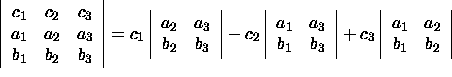Notice that we may now write the formula for the cross product as#### Example

The cross product of the vectors a=<3,-2,-2> and b=<-1,0,5> is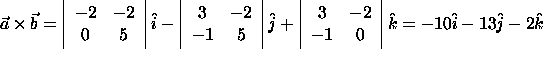Properties of the Cross Product:

• The length of the cross product of two vectors is• The length of the cross product of two vectors is equal to the area of the parallelogram determined by the two vectors (see figure below).
• Anticommutativity:• Multiplication by scalars: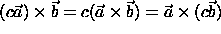• Distributivity: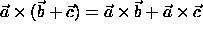• The scalar triple product of the vectors a, b, and c: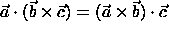• The volume of the parallelepiped determined by the vectors a, b, and c is the magnitude of their scalar triple product.
• The vector triple product of the vectors a, b, and c: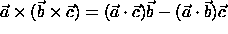Note that the result for the length of the cross product leads directly to the fact that two vectors are parallel if and only if their cross product is the zero vector. This is true since two vectors are parallel if and only if the angle between them is 0 degrees (or 180 degrees).

#### Example

To find the area of the triangle with vertices (1,1,3), (4,-1,1), and (0,1,8), one could find the length of one of the altitudes of the triangle and proceed to find A=1/2(altitude)(base). However, this may not be an easy task in three dimensions. So consider finding the one-half the area of the parallelogram determined by the vector a from (1,1,3) to (4,-1,1) and the vector b from (1,1,3) to (0,1,8). Then a=<2-1,2-(-1),2-3>=<3,-2,-2> and b=<-1,0,5>. Using the result from the previous example and property number two above, we have the length of the cross product (and therefore the parallelogram determined by a and b) as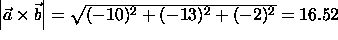So, the area of the traingle is one-half this quantity, or 8.26.

The cross product occurs in many formulas in physics. Some examples include the curl of a vector field (see also Stoke's Theorem), torque, and many integrals over surfaces.